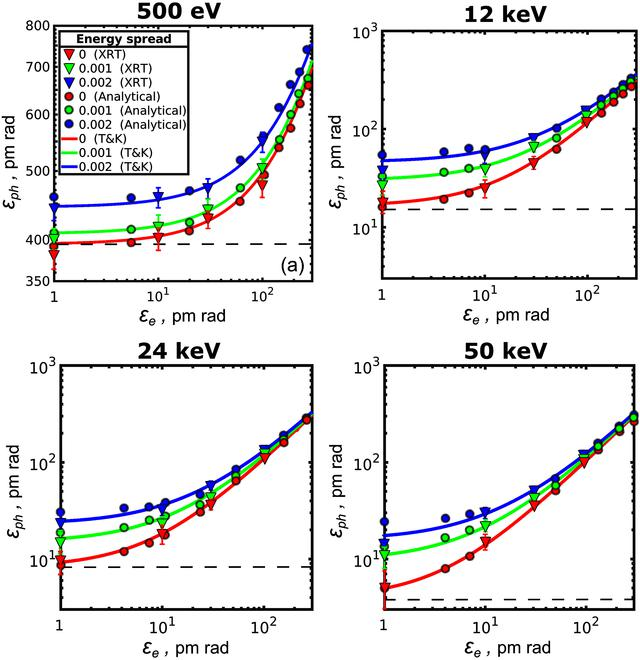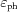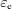disable zoom     view article Figure 1 Photon emittanceas a function of the electron beam emittancefor the different values of the photon energy and energy spread in one transverse direction. Triangles are XRT simulations, circles are analytical calculations and lines are the values obtained from the Tanaka & Kitamura (2009) (T&K) approach [equations (30)and (31)]. Red, green and blue colour correspond to 0, 1 × 10−3 and 2 × 10−3 relative energy spread values, respectively. Note the different scale for the 500 eV emittance value. The dashed horizontal line corresponds to the value of the photon emittance of λ/2π.JOURNAL OFSYNCHROTRONRADIATION
ISSN: 1600-5775
Volume 26| Part 6| November 2019| Pages 1851-1862